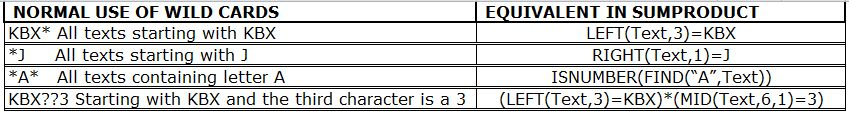#### Email Us

Follows

The 3 wildcard characters (?*~) used in other excel formulas do not work with sumproduct. However, SUMPRODUCT utilizes other functions (LEFT, RIGHT, FIND and MID) to give you the same results.Using below example  showing  Cost Codes and Total Cost,►Find the Sum of the total cost for Sales department if all Sales department cost codes starts with letters “KBX”?

`      =SUMPRODUCT(- -(LEFT(B2:B26,3)="KBX"),C2:C26) = 354,944`

►Find the Sum of the total cost of Stationery if all Stationery cost codes ends with a “J”

`      =SUMPRODUCT(- -(RIGHT(A2:A26,1)="J"),C2:C26) = 353,646`

►Find the total cost of printing for sales department if all printing cost code Starts with KBX and the 6th character is a 3?

`     =SUMPRODUCT(- -(LEFT(A2:A26,3)="KBX")*--(MID(A2:A26,6,1)=3),C2:C26) = 146,454`

### How it Works:

• (LEFT(B2:B26,3)→→creates an array of the 3rd character from the left
`{"KBX";"KBW";"KBW";"KBZ";"KBX";...}`
• LEFT(B2:B26,3)=KBX→→Checks if these characters are equal to KBX and creates a Boolean array
`{TRUE; FALSE; FALSE; FALSE; TRUE;. . .}`
• – –(LEFT(B2:B26,3)=KBX)→→The  Boolean array is converted to its Numbers equivalent by use of double negatives
`{1;0;0;0;1}`
• SUMPRODUCT gets the sum of the product of the two arrays
`({1;0;0;0;1;..},{30360;27520;29240;30260;29660;..})`

### Recommended Tutorial

Beginner to Advanced Excel Course Online

SUMPRODUCT WITH WILD CARDS WORKSHEET